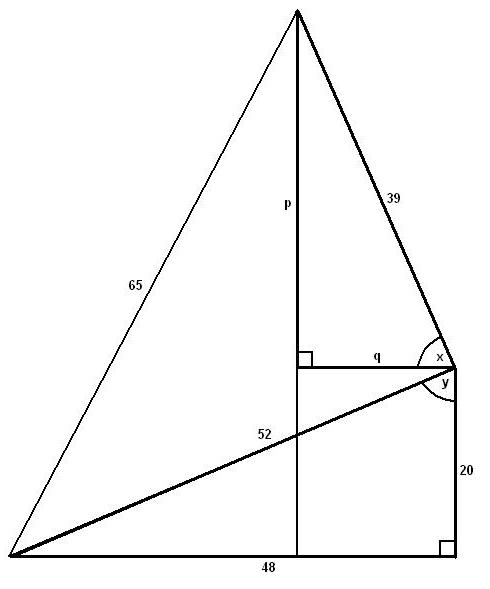#### You may also like### Golden Thoughts

Rectangle PQRS has X and Y on the edges. Triangles PQY, YRX and XSP have equal areas. Prove X and Y divide the sides of PQRS in the golden ratio.### At a Glance

The area of a regular pentagon looks about twice as a big as the pentangle star drawn within it. Is it?### Contact

A circular plate rolls in contact with the sides of a rectangular tray. How much of its circumference comes into contact with the sides of the tray when it rolls around one circuit?

# Wedge on Wedge

##### Age 14 to 16Challenge LevelWe contruct a new triangle as shown. Angle $x$ is equal to $90^\circ - (90^\circ - y) = y$, so the highlighted triangles are similar. Therefore $$p = 48 \times \frac{39}{52} = 36$$ $$q =20\times \frac{39}{52} = 15$$

The base is split $48 - q : q = 33 : 15 = 11 : 5$ and the ball falls a distance of $p + 20 = 36 + 20 = 56$.